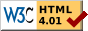## Dokumentation zu: arrays(LPC)``` CONCEPT arrays DESCRIPTION There is support for arrays. Arrays can be declared with the type of its members appended by a star (e.g. string* for an array with string elements). But this declaration is not sufficient to actually create an array at runtime, as all variables (even arrays) are initialized with 0 which is not a valid array. Arrays must either be allocated dynamically with the function 'allocate()' (see efun/allocate), or created with the ({}) array constructor. Arrays are stored by reference, so all assignments of whole arrays will just copy the address. The array will be deallocated when no variable points to it any longer. When a variable points to an array, items can be accessed with indexing: 'arr' as an example. The name of the array being indexed can be any expression, even a function call: 'func()'. It can also be another array, if this array has pointers to arrays: arr = allocate(2); arr = allocate(3); arr = allocate(3); Now 'arr' is a valid value. The 'sizeof()' function (in true C a compiler-directive, not a function) will give the number of elements in an array (see efun/sizeof). NOTE Nowadays it is most of the time preferable to use an array constructor, a list surrounded by '({' and '})', e.g. ({ 1, "xx", 2 }) will construct a new array with size 3, initialized with 1, "xx" and 2 respectively. OPERATIONS INDEXING There are several very useful operations defined on arrays. The most used is the indexing: a=({ 0,1,2,3 }); return a; // this will return 2 You also can count from the end of the array. Use <1 to specify the last element in the array: a=({ 0,1,2,3 }); return a[<3]; // this will return 1 With indexing you can also create sub-arrays: a=({ 0,1,2,3,4,5,6,7 }); return a[3..5]; // this will return ({ 3,4,5 }) return a[2..<2]; // this will return ({ 2,3,4,5,6 }) return a[<5..<3]; // this will return ({ 3,4,5 }) return a[<6..5]; // this will return ({ 2,3,4,5 }) return a[3..3]; // this will return ({ 3 }) return a[3..2]; // this will return ({ }) return a[3..0]; // this will return ({ }) return a[5..100]; // this will return ({ 5,6,7 }) [x..] is interpreted as [x..<1] ADDING You can add two arrays. The result is one array with the elements of both the former arrays: a=({ 0,1 }); b=({ "a","b" }); return a+b; // this will return ({ 0,1,"a","b" }) return b+a; // this will return ({ "a","b",0,1 }) SUBTRACTING You can erase all elements of one array that occur in another array: a=({ 0,1,2,3,4,5,6,7 }); b=({ 7,2,5,8,1,9 }); return a-b; // this will return ({ 0,3,4,6 }) return b-a; // this will return ({ 8,9 }) INTERJUNCTION Use the &-operator to create the interjunction of two arrays: a=({ 5,2,8,1,9,4 }) b=({ 1,6,7,3,4,5 }) return a&b; // this will return ({ 1,4,5 }) ASSIGNING Assigning can also be done to sub-arrays and is thus very powerful: a=({ 0,1,2,3,4,5,6,7 }); a[<4..<3]=({ 8,9 }); return a; // this will return ({ 0,1,2,3,8,9,6,7 }) a=({ 0,1,2,3,4,5,6,7 }); a[2..5]=({ }); return a; // this will return ({ 0,1,6,7 }) a=({ 0,1,2,3,4 }); a[3..2]=({ 8,9 }); return a; // this will return ({ 0,1,2,8,9,3,4 }) a=({ 0,1,2,3,4 }); a[3..0]=({ 8,9 }); return a; // this will return ({ 0,1,2,8,9,1,2,3,4 }) // this is quite funny but true ;-) // WARNING: If done unintentionally and // within a loop, you can quickly cause // the game to run out of memory! GENERAL Of course for any of the operators explained above you can use the combined form of assigning and operating; that means the operators +=, -= and &= work. TIPS If you want to make sure that no element is more than once in an array you can use the following: a = m_indices(mkmapping(a)); This creates a mapping out of the array and recreates the array at once. The elements in the array can be shuffled by this procedure. ```

Start » Magierhandbuch » Docu » LPC » Arrays Letzte Generierung: 25.04.2021, 01:58mud@wl.mud.de